In :
%matplotlib inline

In :
import matplotlib.pyplot as plt
import numpy as np
import pymc3 as pm
import scipy.stats
from sampled import sampled
import seaborn as sns
import theano.tensor as tt

/Users/colin/.venv/bayes_talk/lib/python3.6/site-packages/IPython/html.py:14: ShimWarning: The IPython.html package has been deprecated since IPython 4.0. You should import from notebook instead. IPython.html.widgets has moved to ipywidgets.
"IPython.html.widgets has moved to ipywidgets.", ShimWarning)

In :
# plt.rcParams["figure.dpi"] = 600  # for presentation figures


Linear Regression¶

Define Bayesian linear regression model:

In :
@sampled
def linear_regression(X=None, y=None):
β = pm.Normal('β', mu=0, sd=10, shape=X.shape)
σ = pm.HalfNormal('σ', sd=1)
X = pm.Flat('X', observed=X)
y = pm.Normal('y', mu=X.dot(β), sd=σ, observed=y)


Generate synthetic data, sample from model, and plot posteriors:

In :
X = np.random.normal(size=(100, 4))
β = np.random.normal(size=X.shape)
σ = np.random.normal(size=X.shape)
y = X.dot(β) + σ

with linear_regression(X=X, y=y):
posterior_trace = pm.sample()

Auto-assigning NUTS sampler...
Average Loss = 159.48:   5%|▌         | 10797/200000 [00:00<00:14, 13503.19it/s]
Convergence archived at 12100
Interrupted at 12,100 [6%]: Average Loss = 244.14
100%|██████████| 1000/1000 [00:00<00:00, 1289.89it/s]

In :
ax = sns.distplot(posterior_trace.get_values('σ'))
ax.vlines((σ ** 2).mean(), *ax.get_ylim());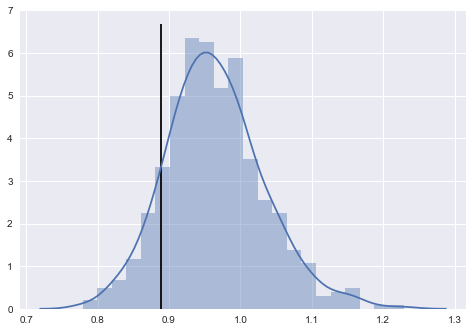In :
fig, axes = plt.subplots(nrows=len(β), sharex=True, sharey=True)

for ax, weight, true in zip(axes, posterior_trace.get_values('β').T, β):
ax = sns.kdeplot(weight.T, ax=ax)
ax.vlines(true, *ax.get_ylim(), alpha=0.5)
ax.set_ylim(bottom=0)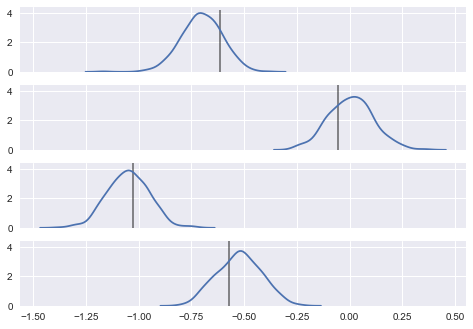Inverting the CDF¶

In :
x = np.linspace(0, 4)
y = 1 - np.exp(-x)
plt.plot(x, y, 'k-', linewidth=6)

z = 0.75
z_inv = -np.log(1 - z)
plt.hlines(z, 0, z_inv, linestyles='dashed', colors='steelblue', linewidth=6)
plt.vlines(-np.log(1 - z), 0, z, linestyles='dashed', colors='steelblue', linewidth=6);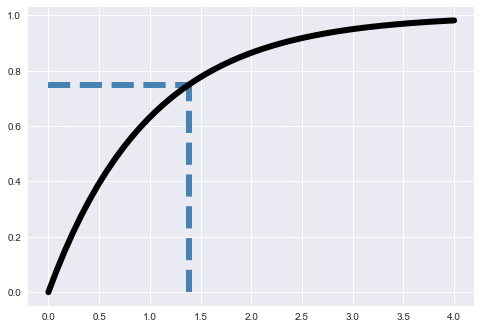Rejection Sampling¶

Here is a pretty general implementation, and a vectorized one for plotting.

In :
def rejection_sampling(pdf, q, k, size):
"""Rejection sampling

Args:
pdf: A (possibly scaled) pdf.  Eats vectors and returns floats
q: A scipy random variable with methods .rv to sample and .pdf to get the pdf of a point
k: A scaling factor to make sure kq(z) >= pdf(z) for all z
size: number of points to sample

Returns:
points: numpy array of sampled points
draws: number of draws it took to get this many points
"""
draws = 0
points = []
while len(points) < size:
proposal = q.rvs(size=1)
draws += 1
accept_prob = pdf(proposal) / (k * q.pdf(proposal))
if accept_prob > 1:
raise RuntimeError('k isn\'t big enough at {}'.format(proposal))
if np.random.random() < accept_prob:
points.append(proposal)
return points, draws

In :
def two_gaussians_pdf():
pdf_one = scipy.stats.norm(2).pdf
pdf_two = scipy.stats.norm(-2).pdf
def pdf(z):
return 0.8 * pdf_one(z) + 0.2 * pdf_two(z)
return pdf

def rejection_sample_plot(N):
"""Hard codes rejection sampling for the above
pdf using a hand-tuned normal distribution
"""
one_d_unknown = two_gaussians_pdf()
one_d_wide = scipy.stats.norm(0, 4)
k = 1.02 * one_d_unknown(2) / one_d_wide.pdf(2)
new_x = one_d_wide.rvs(N)
new_y = np.random.uniform(0, k * one_d_wide.pdf(new_x))
extent = max(abs(new_x.max()), abs(new_x.min()))
if N > 0:
x = np.linspace(min(-8, -1.1 * extent), max(1.1 * extent, 8), 1000)
else:
x = np.linspace(-8, 8, 1000)
fig, ax = plt.subplots()
ax.plot(x, [one_d_unknown(j) for j in x])
ax.plot(x, [k * one_d_wide.pdf(j) for j in x])
ax.fill_between(x, 0, [one_d_unknown(j) for j in x], alpha=0.4)
ax.fill_between(x, 0, [k * one_d_wide.pdf(j) for j in x], alpha=0.1)

accept = new_y <= one_d_unknown(new_x)
ax.plot(new_x[accept], new_y[accept], marker='o', color='green', linestyle='None')
ax.plot(new_x[~accept], new_y[~accept], marker='o', color='red', linestyle='None')

In :
rejection_sample_plot(50)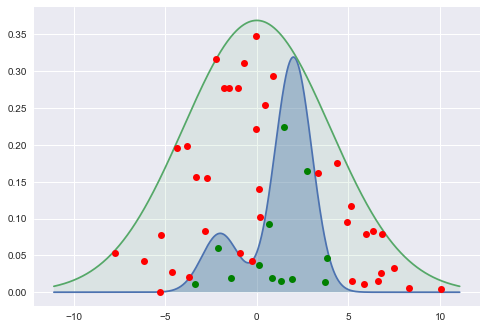Concentration of measure¶

In :
for N in (2, 4, 8, 16, 32):
x = scipy.stats.multivariate_normal(cov=np.eye(N) / N).rvs(size=10000)
_ = sns.distplot(np.linalg.norm(x, axis=1))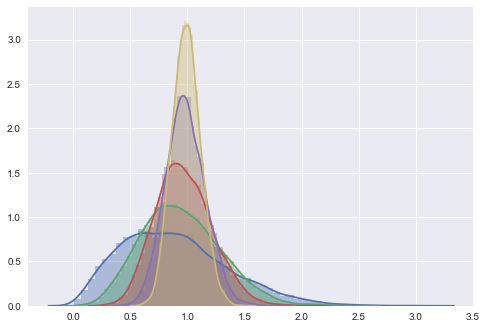Sampling from a donut¶

In :
def jointplot(ary):
"""Helper to plot everything consistently"""
sns.jointplot(*ary.T, alpha=0.1, stat_func=None, xlim=(-1.2, 1.2), ylim=(-1.2, 1.2))

In :
def metropolis_accept(old, new):
"""In log space -- the proposal should be symmetric!"""
return np.log(np.random.uniform()) < new - old

def normal_proposal(scale):
"""Make a proposal for metropolis hastings from the normal distribution."""
def proposal(x):
return np.random.normal(x, scale)
return proposal

def metropolis(logp, n=1000, step_size=0.15, init=np.array([1, 0])):
"""Implementation of Metropolis-Hastings.

The proposal distribution is hardcoded to be a normal distribution,
but it will sample in whatever dimension init is from.  Also prints
the acceptance rate at the end.  Optimal is ~0.234.
"""
proposal = normal_proposal(step_size)
accept = 0
old = proposal(init)
samples = []
for _ in range(n):
new = proposal(old)
if metropolis_accept(logp(old), logp(new)):
old = new
accept += 1
samples.append(old)
print('Acceptance rate: {:.2f}%'.format(100 * accept / n))
return np.array(samples)

In :
def donut_pdf(scale):
"""Sample pdf for visualizations.

Normally distributed around the unit
circle, and the radius may be scaled.
"""
def logp(x):
return -((1 - np.linalg.norm(x)) / scale) ** 2
return logp


Now we actually sample from donut distribution using the handwritten Metropolis sampler above

In :
samples = metropolis(donut_pdf(0.1), n=1000, step_size=0.37)
jointplot(samples)

Acceptance rate: 23.90%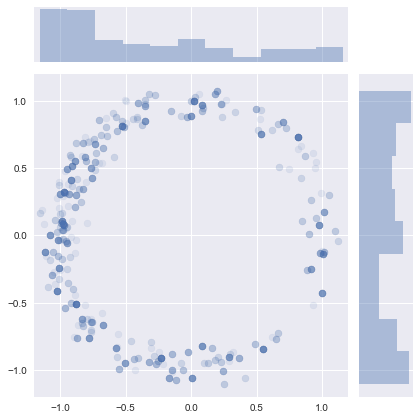Now see how bad the sampler does with a smaller scale on the donut:

In :
samples = metropolis(donut_pdf(0.01), n=1000, step_size=0.03)
jointplot(samples)

Acceptance rate: 25.60%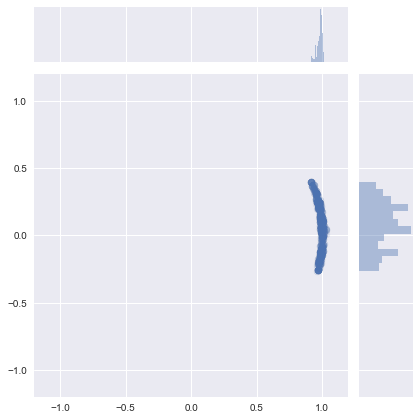We can do the same thing with PyMC3, but we will want the pdf rewritten with theano.

In :
def tt_donut_pdf(scale):
"""Compare to donut_pdf"""
def logp(x):
return -tt.square((1 - x.norm(2)) / scale)
return logp

In :
@sampled
def donut(scale=0.1, **observed):
"""Gets samples from the donut pdf, and allows adjusting the scale of the donut at sample time."""
pm.DensityDist('donut', logp=tt_donut_pdf(scale), shape=2, testval=[0, 1])

In :
with donut(scale=0.1):
metropolis_sample = pm.sample(draws=1000, step=pm.Metropolis())

jointplot(metropolis_sample.get_values('donut'))

100%|██████████| 1500/1500 [00:00<00:00, 8950.14it/s]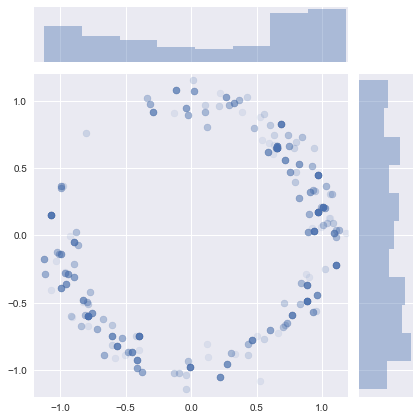PyMC3 also does poorly on a smaller scaled donut (because the problem is the Metropolis sampler, not my implementation!)

In :
with donut(scale=0.01):
metropolis_sample = pm.sample(draws=1000, step=pm.Metropolis())

jointplot(metropolis_sample.get_values('donut'))

100%|██████████| 1500/1500 [00:00<00:00, 9002.16it/s]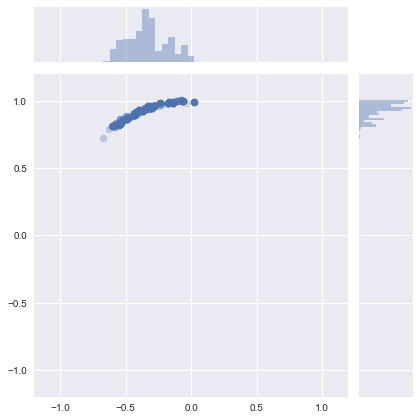Sample the same distributions using the Hamiltonian sampler in PyMC3. It has no problem with either:

In :
with donut(scale=0.1):
nuts_sample = pm.sample(draws=1000, init=None, step=pm.NUTS())

jointplot(nuts_sample.get_values('donut'))

100%|██████████| 1500/1500 [00:02<00:00, 520.08it/s]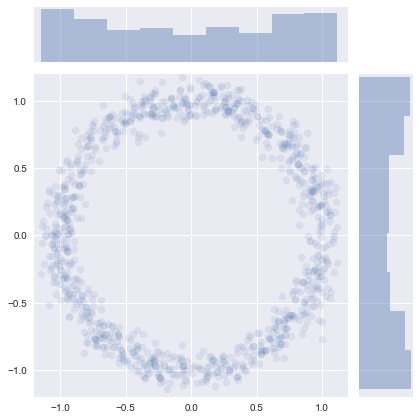In :
with donut(scale=0.01):
nuts_sample = pm.sample(draws=1000, init=None, step=pm.NUTS())

jointplot(nuts_sample.get_values('donut'))

100%|█████████▉| 1493/1500 [00:20<00:00, 63.19it/s]/Users/colin/.venv/bayes_talk/lib/python3.6/site-packages/pymc3/step_methods/hmc/nuts.py:448: UserWarning: Chain 0 reached the maximum tree depth. Increase max_treedepth, increase target_accept or reparameterize.
'reparameterize.' % self._chain_id)
100%|██████████| 1500/1500 [00:20<00:00, 73.96it/s]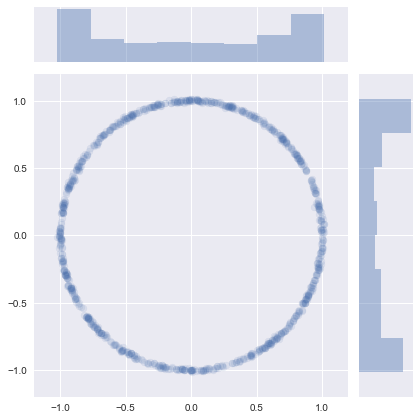Also note that PyMC3 will make good choices if you just call sample:

In :
with donut(scale=0.1) as d:
nuts_sample = pm.sample()

jointplot(nuts_sample.get_values('donut'))

Auto-assigning NUTS sampler...
Average Loss = 23.675:   1%|          | 1929/200000 [00:00<00:10, 19286.52it/s]
Convergence archived at 2200
Interrupted at 2,200 [1%]: Average Loss = 27.687
100%|██████████| 1000/1000 [00:01<00:00, 613.70it/s]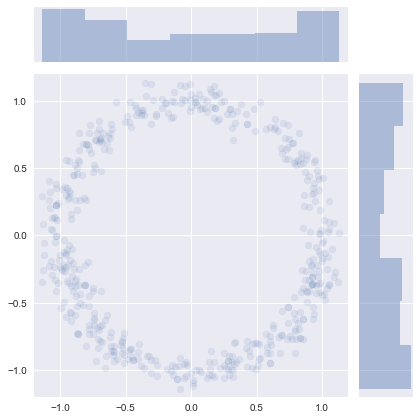$$H(q, p) = -\log \pi(p | q) - \log \pi(q)$$$$\frac{dq}{dt} = \frac{\partial H}{\partial p}$$$$\frac{dp}{dt} = -\frac{\partial H}{\partial q}$$

Example:

Let $\pi(q) = \mathcal{N}(0, 1)$, and $\pi(p | q) = \mathcal{N}(0, 1),$ so $$H(q, p) = C + p^2 + q^2.$$

We can manually compute the gradients for these distributions in order to plot the HMC paths in 2 dimensions: 1 for position, 1 for momentum.

In :
def gen_data(position, momentum, n=10000):
"""Generate a background density plot for the position and momentum distributions.  Not used for sampling."""
q = position.rvs(n)
p = momentum(q).rvs()
return np.column_stack([q, p])

In :
def leapfrog(q, p, dHdq, dHdp, step_size, n_steps):
"""Perform the leapfrog integration.

Similar to the implementations in PyMC3, but
returns an array of all the points in the path
for plotting.  It is a pretty general
implementation, in that it does hardcode
the potential or kinetic energies.

Args:
q: current position
p: current momentum
dHdq: see Hamilton's equations above
dHdp: see Hamilton's equations above
step_size: How big of a step to take
n_steps: How many steps to take

Returns:
(n_steps x 2) numpy array showing the
path through (position, momentum) space
the Hamiltonian path took.
"""
data = [[q, p]]
p += 0.5 * step_size * -dHdq(q, p)
q += step_size * dHdp(q, p)
data.append([q, p])
for _ in range(n_steps - 1):
p += step_size * -dHdq(q, p)
q += step_size * dHdp(q, p)
data.append([q, p])
p += 0.5 * step_size * -dHdq(q, p)
return np.array(data)

In :
def leapfrog_paths(position, momentum, dHdq, dHdp, n=10):
"""Get a number n of paths from the HMC sampler.

This is not quite as general -- I hardcode a step
size of 0.01 and 100 steps, since a path length of 1
looked pretty good (and *not* because it is the best
choice).  Returns an iterator of plot data.
"""
q = position.rvs()
p = momentum(q).rvs()
for _ in range(n):
path = leapfrog(q, p, dHdq, dHdp, 0.01, 100)
yield path
q, _ = path[-1]
p = momentum(q).rvs()


Here we write down the distributions above, and manually compute the gradients needed to use the above two functions.

In :
position = scipy.stats.norm(0, 1)
momentum = lambda q: scipy.stats.norm(0, np.ones(q.shape))
dHdp = lambda q, p: 2 * p
dHdq = lambda q, p: 2 * q

In :
#  First plot a KDE of the joint pdf
X = gen_data(position, momentum)
g = sns.jointplot(*X.T, kind='kde', stat_func=None, xlim=(-3, 3), ylim=(-3, 3), alpha=0.5)

#  Now plot the Leapfrog paths on top of that
for path in leapfrog_paths(position, momentum, dHdq, dHdp, 15):
g.ax_joint.plot(*path.T, linewidth=3)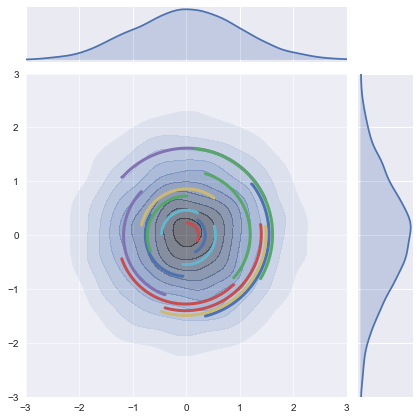Volume of an N-dimensional unit sphere¶

It is pretty small: $$V_n(r) = \frac{\pi^{\frac{n}{2}}}{\Gamma \left(\frac{n}{2} + 1 \right)} r^n$$

In :
from scipy.special import gamma
n = np.linspace(0, 50, 1000)
V = np.power(np.pi, 0.5 * n) / gamma(0.5 * n + 1)
fig = plt.plot(n, V)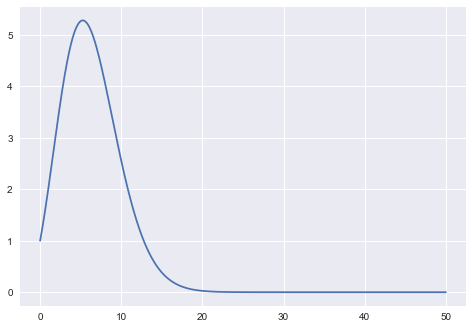In 20 dimensions, how many samples from an enclosing cube fall inside the unit sphere?¶

This actually samples from one "hyper quadrant", but by symmetry, the probability will be the same.

In :
x = np.random.random(size=(20, 10000000))
((x * x).sum(axis=0) < 1).sum()

Out:
0